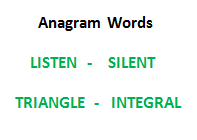# C Program To Check Whether Two Strings Are Anagram Of Each Other

• Last Updated : 20 Dec, 2021

Write a function to check whether two given strings are anagram of each other or not. An anagram of a string is another string that contains the same characters, only the order of characters can be different. For example, “abcd” and “dabc” are an anagram of each other.## We strongly recommend that you click here and practice it, before moving on to the solution.

Method (Count characters):
This method assumes that the set of possible characters in both strings is small. In the following implementation, it is assumed that the characters are stored using 8 bit and there can be 256 possible characters.

1. Create count arrays of size 256 for both strings. Initialize all values in count arrays as 0.
2. Iterate through every character of both strings and increment the count of character in the corresponding count arrays.
3. Compare count arrays. If both count arrays are same, then return true.

Below is the implementation of the above idea:

## C

 `// C program to check if two strings``// are anagrams of each other``#include ``#define NO_OF_CHARS 256`` ` `/* Function to check whether two ``   ``strings are anagram of each other */``bool` `areAnagram(``char``* str1, ``char``* str2)``{``    ``// Create 2 count arrays and initialize ``    ``// all values as 0``    ``int` `count1[NO_OF_CHARS] = {0};``    ``int` `count2[NO_OF_CHARS] = {0};``    ``int` `i;`` ` `    ``// For each character in input strings, ``    ``// increment count in the corresponding ``    ``// count array``    ``for` `(i = 0; str1[i] && str2[i]; i++) ``    ``{``        ``count1[str1[i]]++;``        ``count2[str2[i]]++;``    ``}`` ` `    ``// If both strings are of different length. ``    ``// Removing this condition will make the ``    ``// program fail for strings like "aaca" ``    ``// and "aca"``    ``if` `(str1[i] || str2[i])``        ``return` `false``;`` ` `    ``// Compare count arrays``    ``for` `(i = 0; i < NO_OF_CHARS; i++)``        ``if` `(count1[i] != count2[i])``            ``return` `false``;`` ` `    ``return` `true``;``}`` ` `// Driver code``int` `main()``{``    ``char` `str1[] = ``"geeksforgeeks"``;``    ``char` `str2[] = ``"forgeeksgeeks"``;``   ` `    ``// Function Call``    ``if` `(areAnagram(str1, str2))``        ``printf``(``        ``"The two strings are anagram of each other"``);``    ``else``        ``printf``(``"The two strings are not anagram of each "``               ``"other"``);``    ``return` `0;``}`

Output:

`The two strings are anagram of each other`

Please refer complete article on Check whether two strings are anagram of each other for more details!

My Personal Notes arrow_drop_up